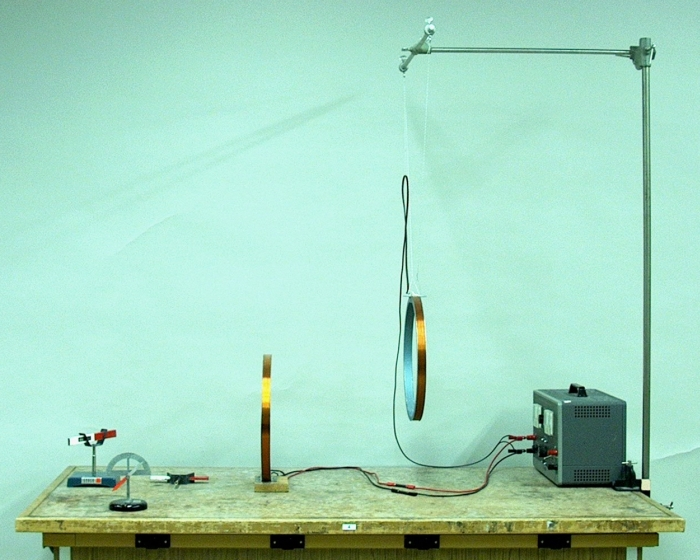(Demonstration idea courtesy of Chuck Reese)

A video of this demonstration is available at this link.

The purpose of this demonstration is to show the direction of the magnetic field lines within the coils, and to impress upon the students the coils’ behavior as electromagnets. With these particular coils, a current of 5 amperes provides a strong enough field to perform the demonstration. At this current, the coils get warm if left for more than about 10 minutes, so it is best not to use larger currents. As shown above, the coils are connected in parallel to the power supply, so the total current provided by the power supply is 10 A.

These coils are short, fat (large-diameter) solenoids. As in any solenoid, the magnetic field lines loop around the coil, and within the coil and next to it along its length, the field lines are parallel to the axis of the coil. (See demonstration 68.13 -- Right-hand rule model.) Thus, when the coils have current flowing in them, they behave as bar magnets, with a north pole on one side and a south pole on the opposite side. You can illustrate this by moving a bar magnet on a swivel all around the coil, inside and outside, or the magnetic dip needle. Removing the base from the magnet on the swivel allows greater freedom in moving it within the coil. When you place the magnet near the open area of the coil, it aligns itself parallel to the coil axis, according to whether it is on the north or south side of the coil.

When the coils are connected as shown and a 5-A current applied to each, when they are facing the same direction, i.e., the north pole of one faces the south pole of the other, the two coils attract each other, and you can pull the hanging coil to one side, perhaps to an angle of about 30 degrees, by bringing the freestanding coil near it. When the two coils point in opposite directions, so that their north poles or south poles face each other, the coils repel. By bringing the freestanding coil next to the hanging coil, you can push the hanging coil aside, to an angle of perhaps as great as 30 degrees, with a gap of about 1/2 inch between the coils. The gap is visible to the students in the lecture hall, so they can see that the coils are actually repelling each other, and that you are not merely supporting one with the other.

As is noted in the explanation for the right-hand rule demonstration mentioned above, the magnetic dipole moment, μ, associated with an electric current flowing in a loop of wire is μ = NiA, where N is the number of turns in the loop, i is the current (in amperes) and A is the area of the loop. By convention, current refers to the flow of positive charge, which is in the opposite direction to the flow of electrons.

The magnetic field around a straight, long wire carrying a current, i, is (1/4πε0c2)(2i/r), where ε0 is the permittivity constant (= 8.854187818 × 10-12 C2/N·m2) and r is the distance from the wire at which you wish to know the magnitude of the field. Because the permeability constant, μ0, = 4π × 10-7 tesla·meter/ampere (=1 henry/meter), 1 tesla = 1 N/C(m/s) = 1 N/(A·m) and 4πε0c2 = 10-7, the magnetic field can also be expressed as (μ0/4π)(2i/r), or (μ0/2π)(i/r), or μ0i/2πr. You can arrive at this via Ampère’s law (∮B · dl = μ0i), integrating over a circular path, l, around the wire, or via the Biot-Savart law (dB = ((μ0i/4π)(dl × r)/r3), finding the contribution to the field at a given point at some distance from the wire, of the current through each element, dl, of the wire, then integrating. The two methods yield the same result, B = μ0i/2πr (in tesla). (1 tesla also equals 1 weber/meter2. The weber, which also equals 1 V·s (= 1 N·m/A, or 1 kg·m2/A·s2), is the unit of magnetic flux.)

If we bend the wire into a loop or coil, consider elements along its circumference, dl, and the magnetic field, dB, due to each of these, and then integrate around the loop, we find that at the center of the loop or coil, the field is B = (μ0/2)(Ni/r), where r is the radius of the loop, and N is the number of turns in the loop (coil). (From the Biot-Savart law, dB = (μ0i/4π)(dl sin θ/r2). Since the current for each element dl is tangent to r, sin θ = 1. Also, dB is in the same direction for each element. All the elements dl sum to 2πr. Integrating around the loop thus gives B = (μ0/2)(Ni/r).)

For points distant from the current loop, B = μ0ir2/2x3, where r is the radius of the loop and x is the distance of the point along the axis perpendicular to the loop. Substituting πR2 for the area of the loop and including the number of turns gives B = (μ0/2π)(NiA/x3), or B = (μ0/2π)(μ/x3).

References:

1) Feynman, Richard P., Leighton, Robert B. and Sands, Matthew. The Feynman Lectures on Physics (Menlo Park, California: Addison-Wesley Publishing Company, 1963) Volume II, section 13-5.
2) Halliday, David and Resnick, Robert. Physics, Part Two, Third Edition (New York: John Wiley and Sons, 1978), pp. 727, 747-750, 758-762.
3) Sears, Francis Weston and Zemansky, Mark W. College Physics (Reading, Massachussetts: Addison-Wesley Publishing Company, Inc., 1960), pp. 641-8.